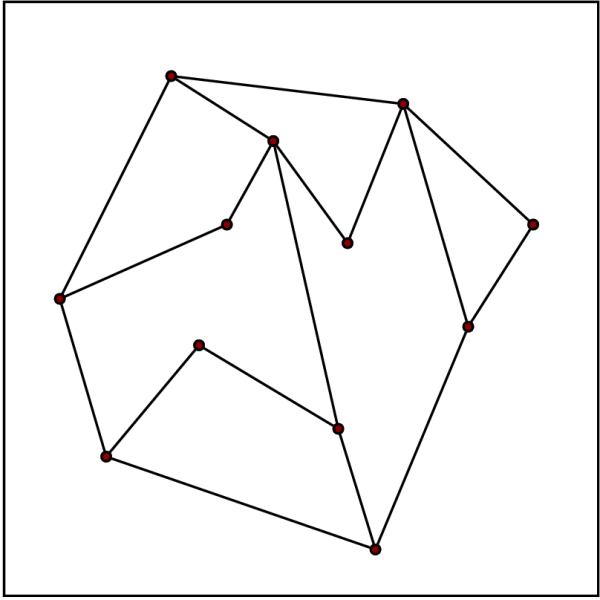# Planar straight line graphs (PSLGs) in Data Structure

Data Structure AlgorithmsAnalysis of AlgorithmsAlgorithms

In case of computational geometry, a planar straight-line graph, in short PSLG, (or straight-line plane graph, or plane straight-line graph) is defined as a term implemented for an embedding of a planar graph in the plane such that its edges are mapped into straight line segments. Statement of Fáry's theorem (1948) is that every planar graph has this kind of embedding.

In case of computational geometry, PSLGs have often been termed planar subdivisions, with an assumption or assertion that subdivisions are polygonal.

Without vertices of degree 1, a PSLG defines a subdivision of the plane into polygonal regions and vice versa. Absence of degree 1 vertices simplifies descriptions of various algorithms, but it is not essential.

Representations of various maps may be served by PSLGs, e.g., geographical maps in geographical information systems.

PSLGs have some special cases. The special cases are triangulations (polygon triangulation, point set triangulation). The point set triangulations are maximum PSLGs in the sense that it is not possible to add straight edges to them while keeping the graph planar. The triangulations have various applications in so many areas.

The PSLGs can be seen as a special kind of Euclidean graphs. Moreover, in discussions involving Euclidean graphs, the main interest is their metric properties, i.e., distances between vertices, while for PSLGs the main interest is the topological properties. In case of some graphs, such as Delaunay triangulations, both metric and topological properties are of importance.## Representations

PSLGs are represented by three well-known data structures. These data structures are the Winged-edge data structure, Halfedge, and Quadedge. Winged-edge data structure is the oldest of the three, but manipulating it often needs complicated case distinctions. The reason behind that edge references do not store the edge direction, and the directions of edges around a face need not be consistent. Halfedge data structure is useful to store both orientations of an edge and links them properly, simplifying operations and the storage scheme. Quadedge data structure is useful to store both the planar subdivision and its dual simultaneously. Records of it consist explicitly only of edge records, four for each edge, and in a simplified form it is useful for storing PSLGs.